## General Question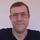# What's the longest known sequence of non-prime numbers?

Asked by mattbrowne (31671) February 12th, 2012

Here’s what I mean:

Length 3:
8,9,10
14,15,16
20,21,22

Length 5:
24,25,26,27,28

Observing members: 0Composing members: 0My guess would be that such sequences grow in length with as number size gets larger. Prime numbers become increasing scarce as numbers get larger, making it more likely to find long sequences of primes.

LostInParadise (29152)“Great Answer” (5) Flag as…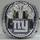bkcunningham (18461)“Great Answer” (4) Flag as…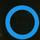The longest I see in numbers up to one thousand is nineteen:
888, 889, 890, 891, 892, 893, 894, 895, 896, 897, 898, 899, 900, 901, 902, 903, 904, 905, 906
Beyond that I don’t know.

amujinx (5170)“Great Answer” (3) Flag as…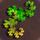Here’s a neat proof to show why there cannot be a maximum gap between primes-

First, you must know that X*Y+X is always divisible by X and thus not prime.

Take a number N, and compute the factorial of it. The factorial of N (symbolized as N!) is the product of all of the natural numbers less than or equal to N, excluding one.
5! = 5*4*3*2 = 120
For any number in this set, 120 can be written as Number*AllOtherNumbersInSet. For example, 120 can be written as 4*(2*3*5), or maybe 2*(3*4*5).

This is the neat part-
122 can be written as 2*(3*4*5)+2, so it is composite.
123 can be written as 3*(2*4*5)+3, so it is composite.
124 can be written as 4*(2*3*5)+4, so it is composite.
125 can be written as 5*(2*3*4)+5, so it is composite.

Extending this principle past 5! means that for any number N, all numbers N!+2 through N!+N are composite, always creating a sequence of N-1 composite numbers. By picking larger numbers for N, you can always find a larger gap!

PhiNotPi (12677)“Great Answer” (9) Flag as…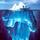@PhiNotPi Nice !!

gasman (11315)“Great Answer” (1) Flag as…I agree! Just to show how strange primes are, n! + 1 is either a prime or else all of its prime factors are greater than n, since division by any number less than or equal to 1 leaves a remainder of 1.

LostInParadise (29152)“Great Answer” (1) Flag as…This reminds me of Euclid’s proof of the infinitude of primes. Let P equal the product of the first n primes, p1*p2*...*pn. Then P + 1 is divisible by a prime greater than pn. The numbers P+2, P+3,...,P+pn are all non-prime.

LostInParadise (29152)“Great Answer” (1) Flag as…Great answers. Thank you! 1,442 is an impressive length for a prime gap.

The theorem that for any natural number, n, there exists a set of n consecutive integers such that none are prime is still somewhat puzzling. Although there’s an infinite number of prime numbers, there’s also no limit for prime gaps. Wow.

Well, at first the set of rational numbers seems larger than natural numbers as well.

mattbrowne (31671)“Great Answer” (0) Flag as…Matt, You may find this article on the Prime Number Theorem Note that the proportion of primes less than x = pi(x)/x, which is of the order 1/ln(x). The limit of 1/ln(x) is zero, but it gets there really slowly.

That the proportion of primes decreases is not hard to see. ½ of all numbers up to x are divisible by 2. ⅓ are divisible by 3. The proportion divisible by 2 or 3 is ½ + ⅓ – ½*⅓ = ⅔, so the proportion of primes up to x must be less than ⅓. We can keep subtracting from this proportion as we increase the number of primes.

LostInParadise (29152)“Great Answer” (1) Flag as…Well, what does this mean in terms of prime gaps? Finding a sequence of 1442 non-prime numbers required a search up to 10^18. When can we expect a 2884 prime gap? What would the formula look like if m is the length of the prime gap and n is the numbers we must search?

mattbrowne (31671)“Great Answer” (0) Flag as…Good questions but beyond my capability and perhaps beyond what we are currently able to determine, beyond coming up with very large upper bounds for the occurrence.

LostInParadise (29152)“Great Answer” (1) Flag as…The simplest upper bound on a 2884 prime gap will be found starting on 2885!+2 and ending on 2885!+2885. Those are truly massive numbers, and I wouldn’t be surprised if it was found much earlier than that. Actually, it is guaranteed to happen much earlier than that.

Instead of multiplying every number less than or equal to a given number, you can only multiply the prime numbers less than or equal to that number. This will produce a much, much lower upper bound. The downside is that computing this upper bound requires knowledge of all of the primes less than or equal to that number.

PhiNotPi (12677)“Great Answer” (3) Flag as…Thanks @PhiNotPi !

mattbrowne (31671)“Great Answer” (0) Flag as…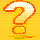This is essentially a question about Prime Gaps. It has been extensively studied but I don’t think a formula for the first pair of primes with a gap larger than a given number n has been found yet.
http://en.wikipedia.org/wiki/Prime_gap

BonusQuestion (1483)“Great Answer” (1) Flag as…or